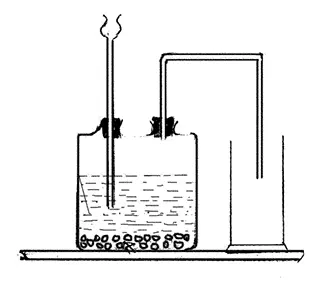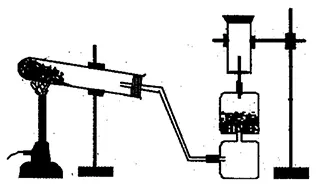# 3 and 4 Marks Question

1. Study the given diagram and answer the following questions.1. Which gas is collected in gas jar?
2. CO2 gas is collected in gas jar.
3. Why is the gas jar kept erect?
4. The gas jar is kept erect because CO2 is heavier than air.
5. What happen when gas is reacted with potassium hydroxide? Write the balanced chemical equation.
6. When gas is reacted with potassium hydroxide, potassium carbonate along with water is formed.
CO2 + KOH ⟶ K2CO3 + H2O
CO2 + 2KOH ⟶ K2CO3 + H2O
2. Draw a neat diagram to show the laboratory preparation of carbon dioxide gas and label any three parts.
3. Carbon dioxide gas is produced in lab by the chemical reaction between dilute hydrochloric acid and marble pieces.
CaCO3 + HCl ⟶ CaCl2 + H2O + CO2
4. Which gasses are produced when ammonium carbonate is heated in a hard-glass test tube? Write the balanced chemical equation of the chemical reaction that takes place in the hard-glass test tube when ammonium carbonate is heated.
5. Carbon dioxide and ammonia gases are produced when ammonium carbonate is heated in a hard glass test tube.

(NH4)2CO3 $\xrightarrow{\triangle}$ NH3 + CO2 + H2O
(NH4)2CO3 $\xrightarrow{\triangle}$ 2NH3 + CO2 + H2O

6. How can we prepare a weak acid from carbon dioxide? Write with chemical equation.
7. We can prepare a weak acid from carbon dioxide by reacting it with water.
CO2 + H2O ⟶ H2CO3 (Carbonic acid)
8. Study the given diagram and answer the following questions.1. Which gas is collected in jar?
2. Ammonia gas is collected in jar.
3. Why is lime tower used to collect the gas?
4. CaO in lime tower absorbs the water and helps to obtain pure and dry ammonia. So, the lime tower was used to collect the gas.
5. What happens when this gas is passed in water? Write chemical reaction.
6. Ammonium hydroxide is formed when this gas is passed into water. NH3 + H2O ⟶ NH4OH
9. Draw a diagram of laboratory preparation of ammonia gas and label any four parts. Why this gas can not be collected in the gas jar by downward displacement of water? Write one use of it.
11. Study the given diagram and answer the following questions.1. Which gas is being collected in gas jar?
2. Ammonia gas is being collected in gas jar.
3. Why is the mouth of the hard glass test tube inclined downward?
4. The mouth of the hard glass test tube inclined downward to prevent the water so formed during the reaction to drop at the hot bottom of the the tube which may cause a burst of the tube.
5. What happen when sulphuric acid is reacted with the gas formed? Write balanced chemical equation.
6. When sulphuric acid is reacted with the gas formed ammonium sulphate is formed.
NH3 + H2SO4 ⟶ (NH4)2SO4
2NH3 + H2SO4 ⟶ (NH4)2SO4
12. How does the ammonia gas is manufactured on industrial scale? Write with balanced chemical equation.
13. The ammonia gas is manufactured on industrial scale by combination reaction of nitrogen and hydrogen in the ratio of 1:3 at 2050 atm pressure, 500°C temperature, using powder of iron as catalyst and Molybdenum as promoter.
N2 + 3H2 $\xrightarrow[500\degree C/250 Atm]{Fe/Mo}$ 2NH3
14. Study the given diagram and answer the following questions.1. Which gas is collected in gas jar?
2. Carbon dioxide gas is collected in gas jar.
3. This gas cannot be collected by downward displacement of water. Why?
4. This gas cannot be collected by downward displacement of water because it is soluble in water (reacts with water and form weak acid).
5. How can you manufacture urea from the gas collected in gas jar? Write with balanced chemical equation.
6. We can manufacture urea from the gas collected in a gas jar by reacting it with ammonia under pressure and at about 1500°C temperature.
CO2 + NH3 $\xrightarrow{1500\degree C}$ NH2CONH2 + H2O
CO2 + 2NH3 $\xrightarrow{1500\degree C}$ NH2CONH2 + H2O
15. Draw a diagram of laboratory preparation of carbon dioxide gas. Name two chemicals used for this process.

Reaction:
CaCO3 + HCl ⟶ CaCl2 + H2O + CO2
CaCO3 + 2HCl ⟶ CaCl2 + H2O + CO2

Chemicals that are required to prepare carbon dioxide gas in the laboratory are: dilute hydrochloric acid (HCl), marble pieces (CaCO3).

17. Study the given diagram and answer the following questions.1. Which gas is collected in gas jar?
2. Ammonia gas is collected in gas jar.
3. Which colored litmus paper is used to identify such gas?
4. Red colored litmus paper is used to identify such gas.
5. Why does this gas is collected in inverted gas jar?
6. This gas is collected in inverted gas jar because it is lighter than air.
7. What happens when lime tower is not used to collect such gas?
8. CaO in lime tower absorbs the water and helps to obtain pure and dry ammonia. If the lime tower is not used to collect such gas moist ammonia will be obtained.
18. According to the given figure, which gas is being collected in the gas jar? Write the balanced chemical equation of the reaction in the preparation of the gas. Why is the gas not collected in the gas jar by the downward displacement of water?19. According to the given figure, ammonia is being collected in the gas jar. Write the balanced chemical equation of the reaction in the preparation of the gas.
It is prepared by heating the mixture of ammonium chloride and calcium hydroxide.

NH4Cl + Ca(OH)2 $\xrightarrow{\triangle}$ CaCl<sub

2</sub

• H2O + NH3
2NH4Cl + Ca(OH)2 $\xrightarrow{\triangle}$ CaCl<sub

2</sub

• 2H2O + 2NH3
The gas not collected in the gas jar by the downward displacement of water because it is highly soluble in water and forms ammonium hydroxide.
NH3 + H2O ⟶ NH4OH
20. Answer the following questions based on the given diagram.1. Which gas is being collected in the gas jar?
2. Carbon dioxide is being collected in the gas jar.
3. What happens when some lime-water is put in the gas jar?
4. When some lime water is put in the gas jar for short time it turns to milky solution due to the formation of insoluble calcium carbonate.

Ca(OH)2 + CO2 ⟶ CaCO3 ↓ + H2O

Similarly, if the carbon dioxide is continued to pass for a long time, it turns into colorless due to formation of solution sodium bicarbonate.

CaCO3 + H2O + CO2 ⟶ Ca(HCO3)2

5. Write down the balanced chemical equation of the chemical reaction in the formation of the given gas.
6. Carbon dioxide gas is produced by the chemical reaction between dilute hydrochloric acid and marble pieces.
CaCO3 + HCl ⟶ CaCl2 + H2O + CO2
CaCO3 + 2HCl ⟶ CaCl2 + H2O + CO2